# Graphs in MATLAB R2015b

I was inspired by ICIP 2015 and by the new graph functions in MATLAB R2015b to write some functions to help experiment with graph-based image processing algorithms. Earlier this week I submitted the code to the File Exchange. Here's a screen shot:Over the next couple of weeks I want to show you how to get started with these functions. Today starts with a general introduction to graphs in MATLAB R2015b. I'll be picking on the state of Minnesota.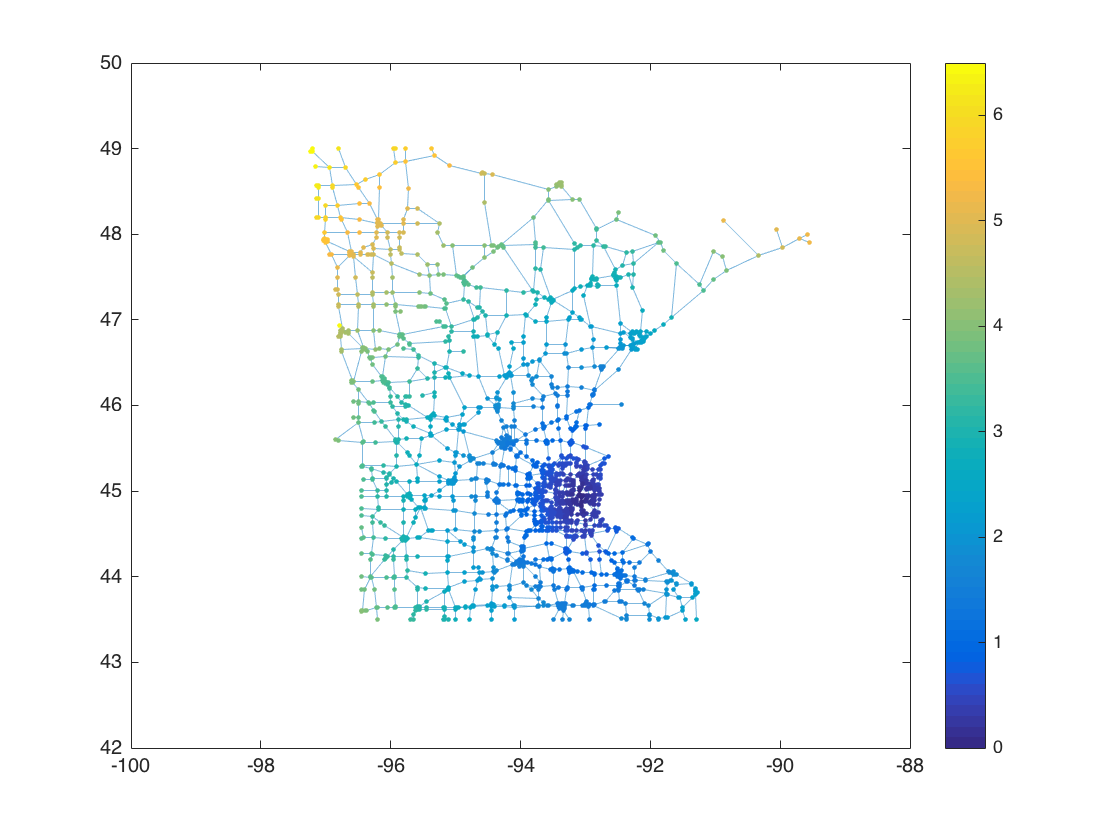There are lots of ways to create a graph. A simple way is to provide two lists of nodes. Each corresponding pair of nodes corresponds to an edge. Nodes can be numbered or identified by text labels.

G = graph({'A', 'B', 'B'}, {'B', 'C', 'D'})

G =

graph with properties:

Edges: [3x1 table]
Nodes: [4x1 table]



As shown above, a graph contains a table of nodes and a table of edges.

You can use the plot function to visualize a graph.

plot(G)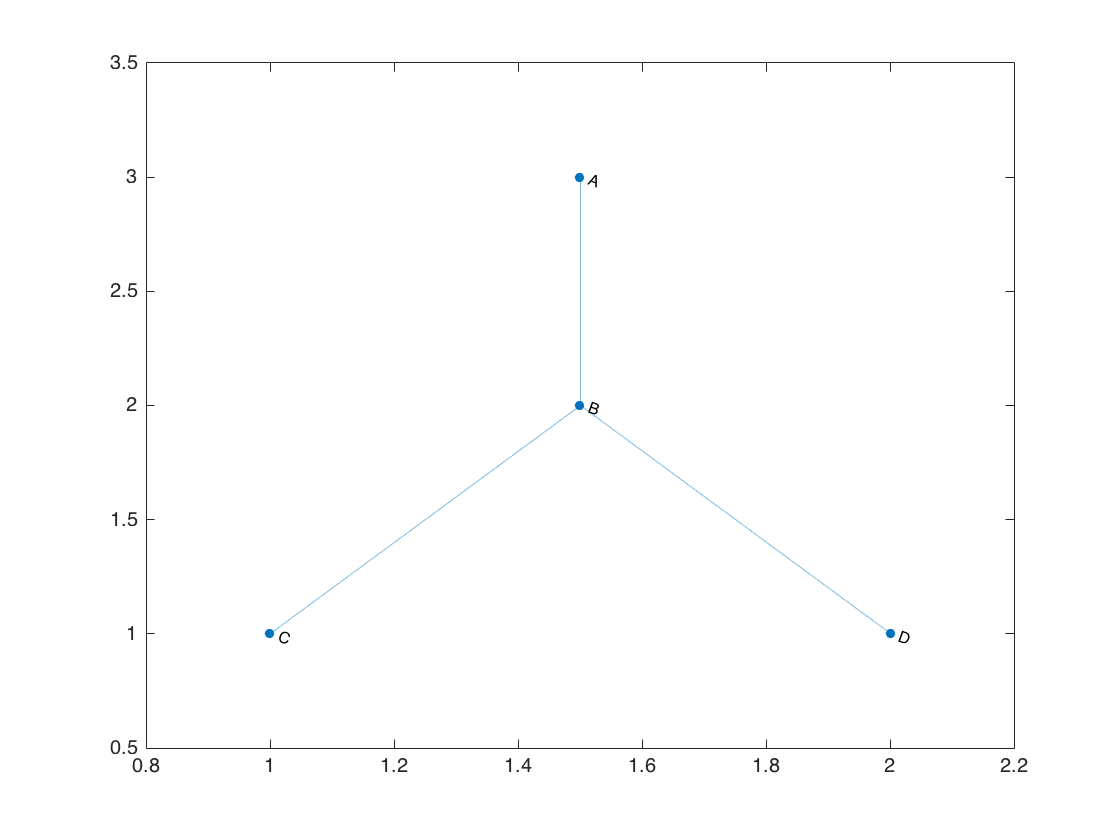Use digraph to make a directed graph. Here's one that uses node numbers,node names, and edge weights.

s = [1 1 2 2 3 3 4 4 5 5];
t = [2 5 3 4 4 5 3 1 1 6];
names = {'alpha', 'beta', 'gamma', 'delta', 'epsilon', 'zeta'};
weight = randi(100, size(s));
D = digraph(s, t, weight, names)

D =

digraph with properties:

Edges: [10x2 table]
Nodes: [6x1 table]



The weight values are stored directly in the edge table.

D.Edges

ans =

EndNodes           Weight
______________________    ______

'alpha'      'beta'       24
'alpha'      'epsilon'    36
'beta'       'gamma'      83
'beta'       'delta'       2
'gamma'      'delta'       5
'gamma'      'epsilon'    17
'delta'      'alpha'      74
'delta'      'gamma'      65
'epsilon'    'alpha'      65
'epsilon'    'zeta'       46



When you plot a directed graphs, arrows show the edge directions.

plot(D)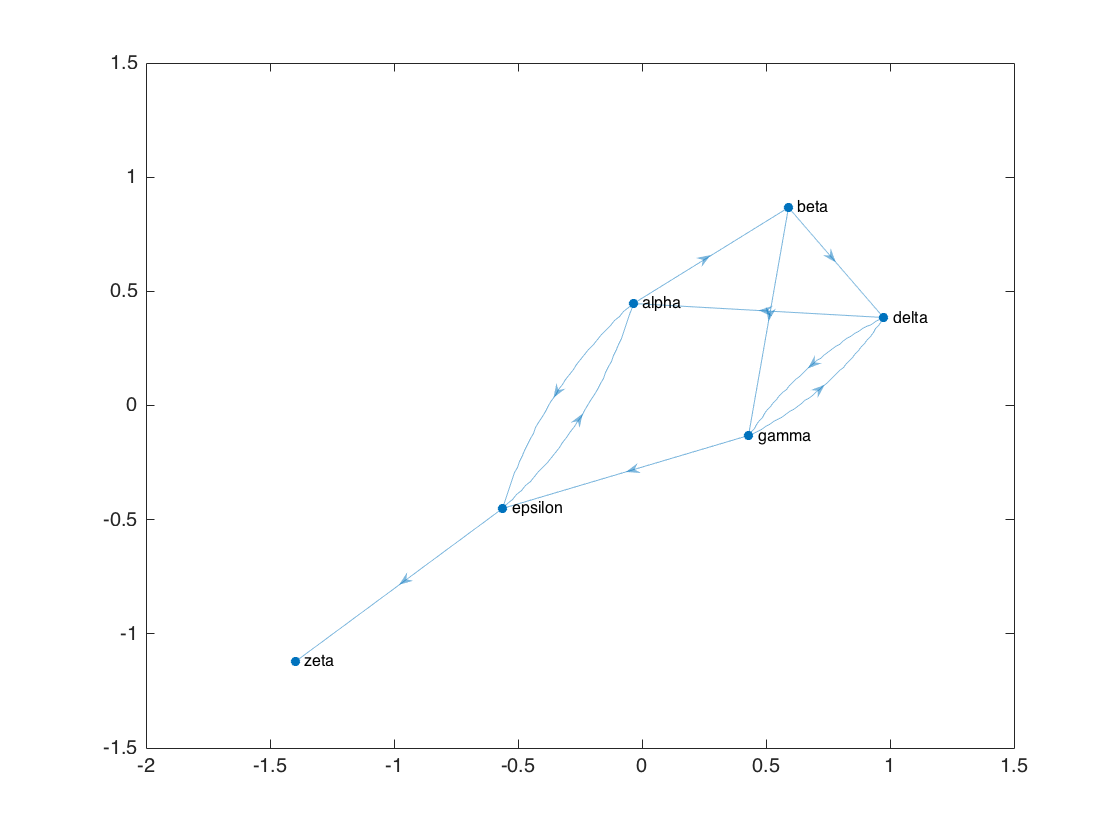You can choose different plot layouts.

plot(D,'Layout','layered')
title('Layered graph plot')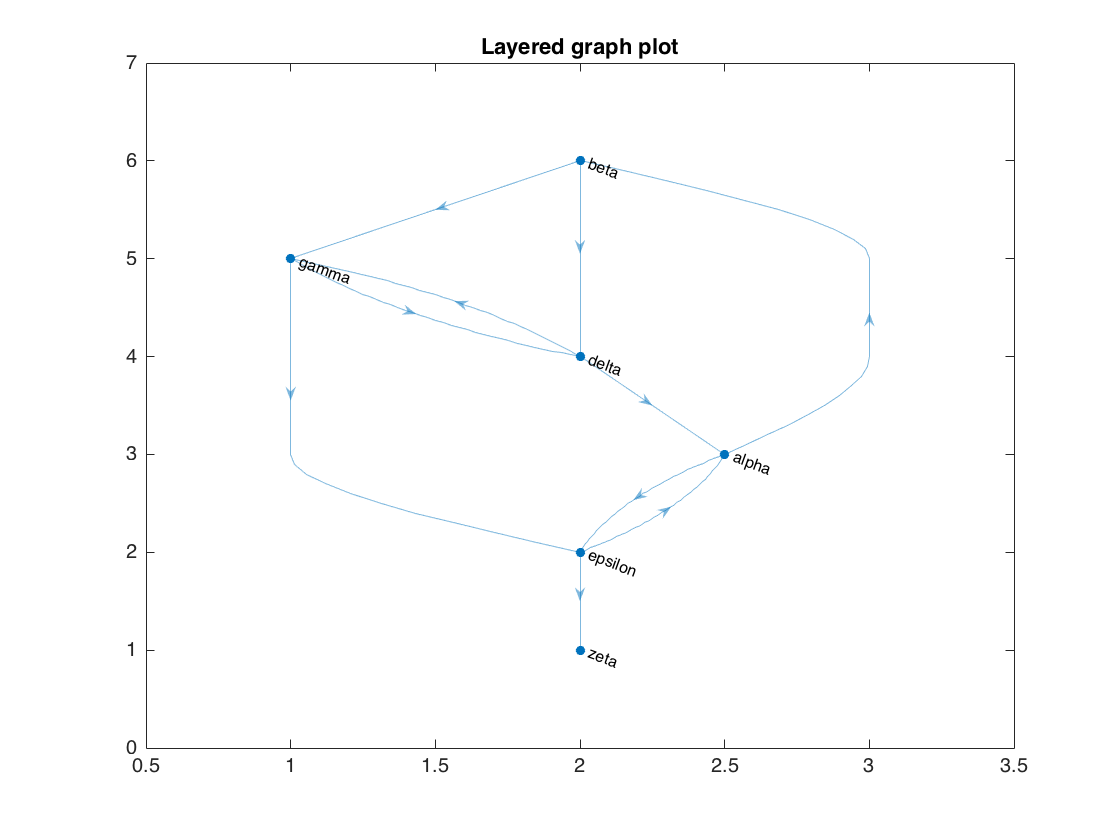plot(D,'Layout','circle')
axis equal
title('Circular graph plot')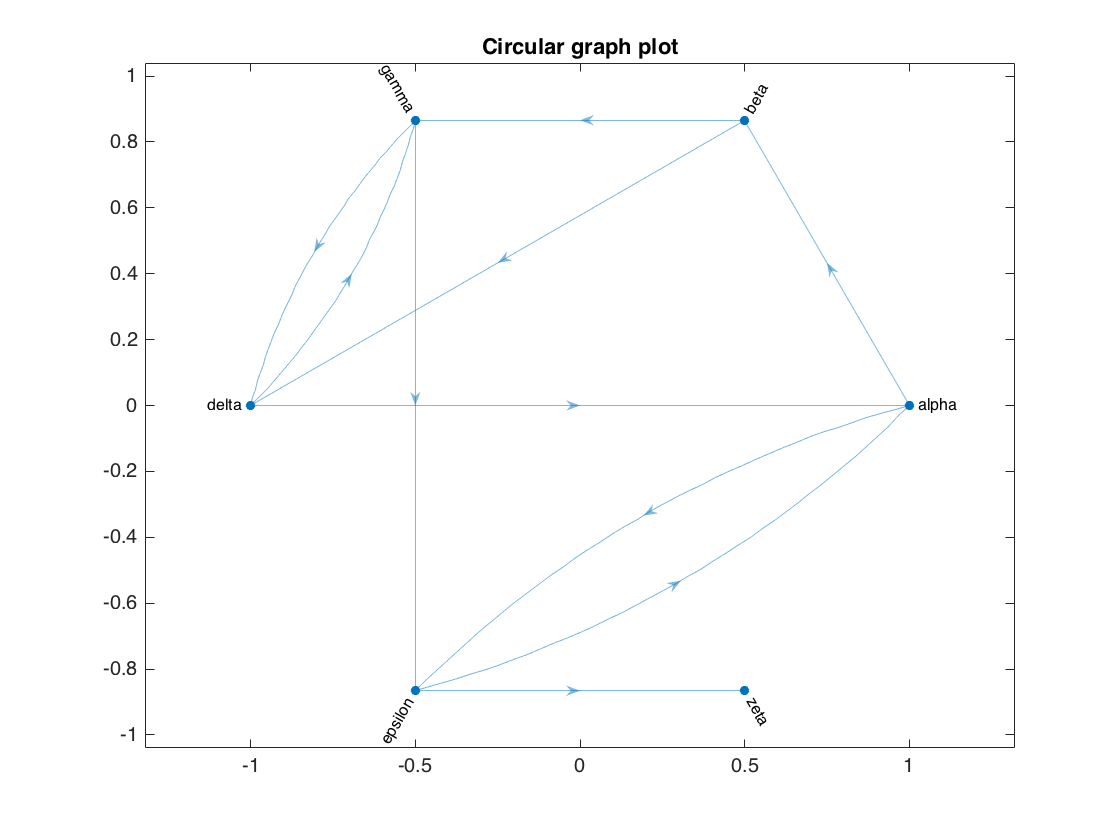You can customize a GraphPlot directly. Call the plot function with an output argument to get the GraphPlot object.

p = plot(D)

p =

GraphPlot with properties:

NodeColor: [0 0.4470 0.7410]
MarkerSize: 4
Marker: 'o'
EdgeColor: [0 0.4470 0.7410]
LineWidth: 0.5000
LineStyle: '-'
NodeLabel: {'alpha'  'beta'  'gamma'  'delta'  'epsilon'  'zeta'}
EdgeLabel: {}
XData: [-0.0350 0.5891 0.4312 0.9742 -0.5613 -1.3981]
YData: [0.4486 0.8689 -0.1321 0.3850 -0.4499 -1.1206]

Use GET to show all properties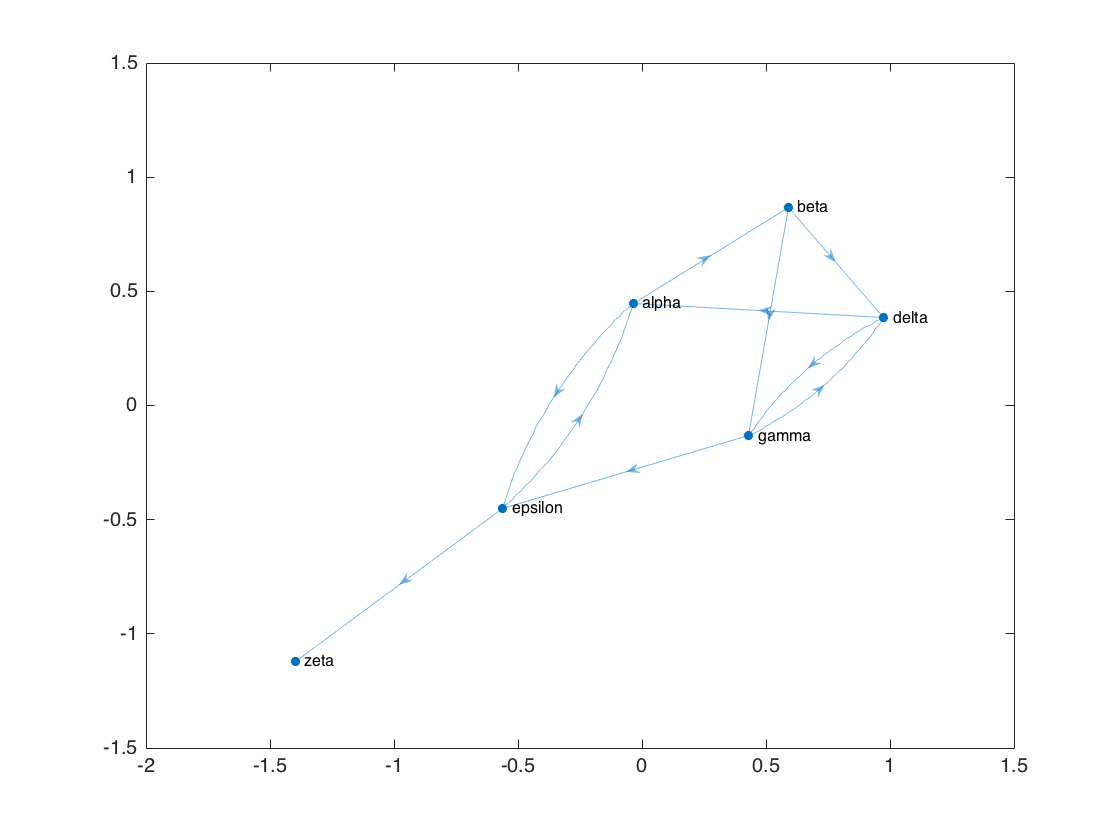Now modify some of the properties.

p.MarkerSize = 8;
p.Marker = 'x';
p.EdgeColor = 'red';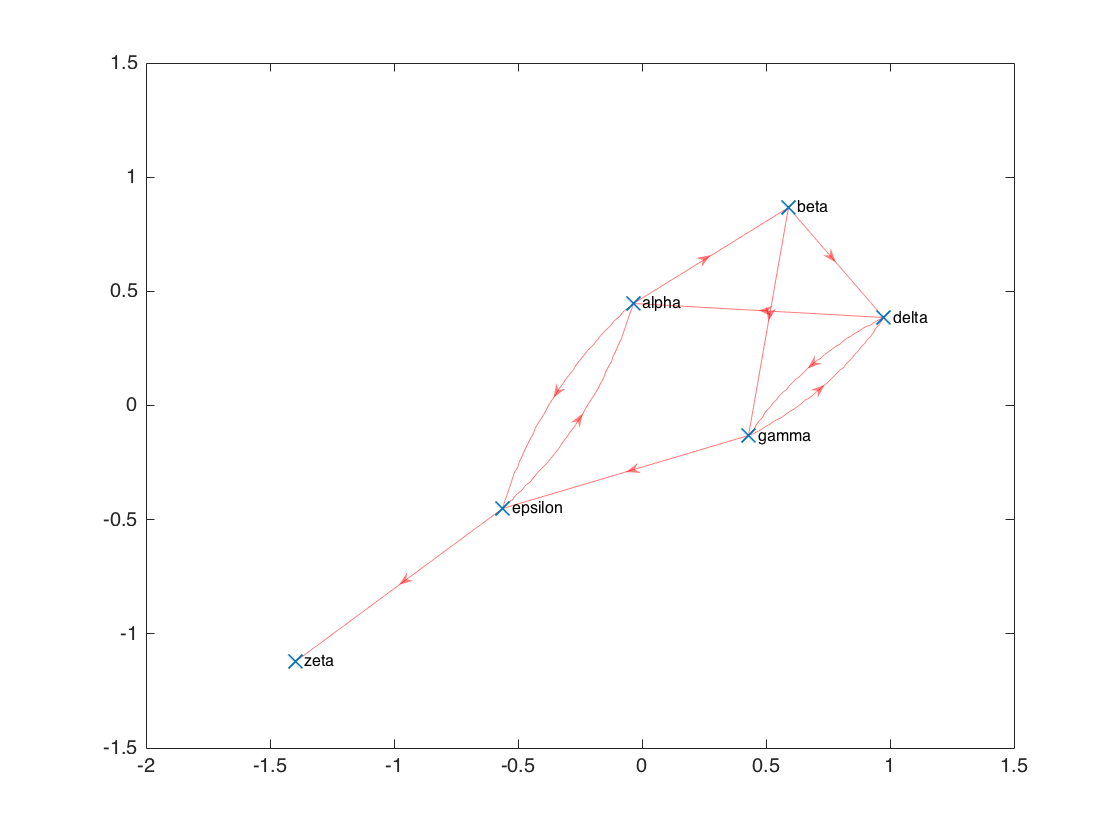I really like the highlight function, which you can use to emphasize selected graph nodes and edges.

Here I highlight the shortest path between nodes 'alpha' and 'zeta'.

p = plot(D);
shortPath = shortestpath(D, 'alpha', 'zeta')
highlight(p, shortPath)

shortPath =

'alpha'    'epsilon'    'zeta'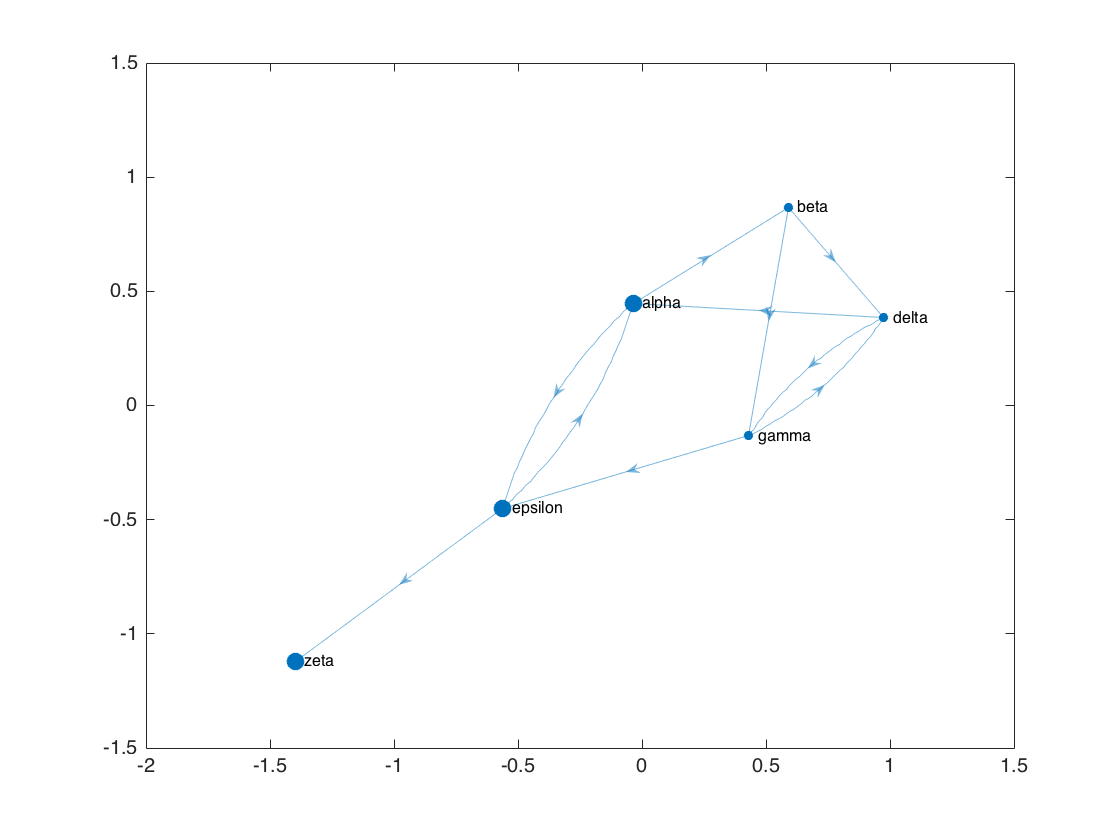Many graph computations are available. For example, you can compute the pair-wise weighted distance between all nodes, or the out-degree of all nodes.

distances(D)

ans =

0    24    91    26    36    82
76     0    67     2    84   130
79   103     0     5    17    63
74    98    65     0    82   128
65    89   156    91     0    46
Inf   Inf   Inf   Inf   Inf     0



Poor node 6. You can't get anywhere else from there! That's because its out-degree is 0.

outdegree(D)

ans =

2
2
2
2
2
0



Let's look at a somewhat larger graph, based on the network of roads in the state of Minnesota. (If you want to play with this data yourself, you can get it from the UF Sparse Matrix Collection.)

load('minnesota.mat')
xy = Problem.aux.coord;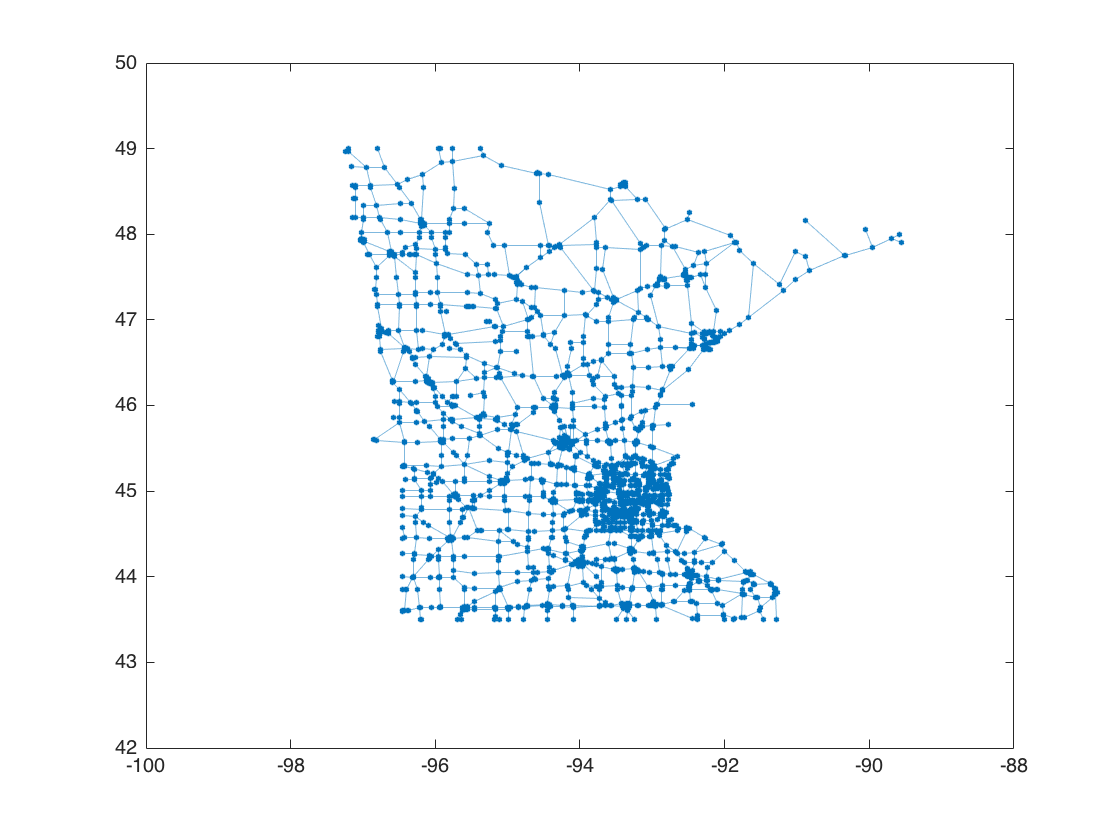You can compute a graph's minimum spanning tree.

T = minspantree(minn_roads)
plot(T,'XData',xy(:,1),'YData',xy(:,2));

T =

graph with properties:

Edges: [2639x2 table]
Nodes: [2642x0 table]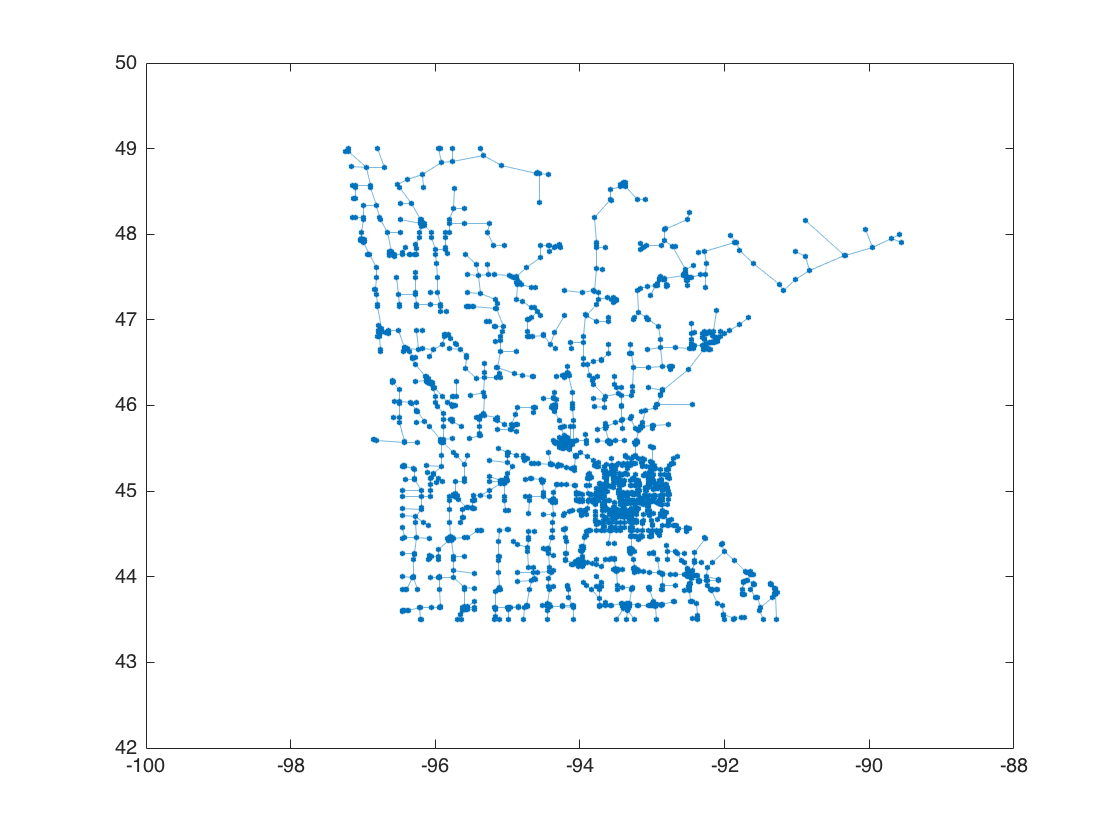This example shows how to color the graph by the shortest path tree.

[tree, d] = shortestpathtree(minn_roads, 1561);
p.NodeCData = d;
colorbarNext time I'll start talking about graphs whose nodes are image pixels.

Published with MATLAB® R2015b

|